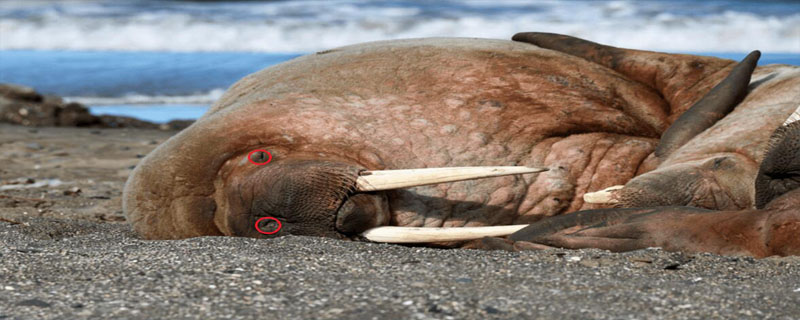# python中的海象运算符## 1. 第一个用法：if/else

```import "fmt"

func main() {
if age := 20;age > 18 {
fmt.Println("已经成年了")
}
}```

```age = 20
if age > 18:
print("已经成年了")```

```if (age:= 20) > 18:
print("已经成年了")```

## 2. 第二个用法：while

```file = open("demo.txt", "r")
while True:
if not line:
break
print(line.strip())```

```file = open("demo.txt", "r")
print(line.strip())```

```while True:
p = input("Enter the password: ")
break```

```while (p := input("Enter the password: ")) != "youpassword":
continue```

## 3. 第三个用法：推导式

```members = [
{"name": "小五", "age": 23, "height": 1.75, "weight": 72},
{"name": "小李", "age": 17, "height": 1.72, "weight": 63},
{"name": "小陈", "age": 20, "height": 1.78, "weight": 82},
]

count = 0

def get_bmi(info):
global count
count += 1

print(f"执行了 {count} 次")

height = info["height"]
weight = info["weight"]

return weight / (height**2)

# 查出所有会员中过于肥胖的人的 bmi 指数
fat_bmis = [get_bmi(m) for m in members if get_bmi(m) > 24]

print(fat_bmis)```

```执行了 1 次

[25.88057063502083]```

```fat_bmis = []

# 查出所有会员中过于肥胖的人的 bmi 指数
for m in members:
bmi = get_bmi(m)
if bmi > 24:
fat_bmis.append(bmi)```

```# 查出所有会员中过于肥胖的人的 bmi 指数
fat_bmis = [bmi for m in members if (bmi := get_bmi(m)) > 24]```

```执行了 1 次

[25.88057063502083]```

Python开发开发项目